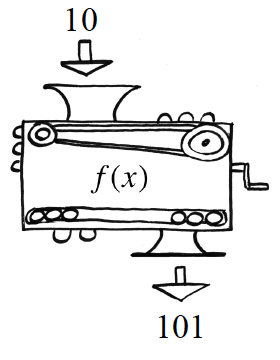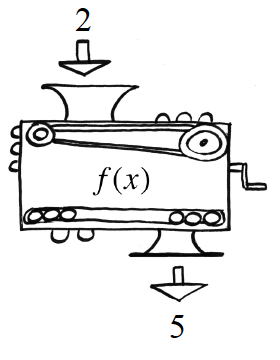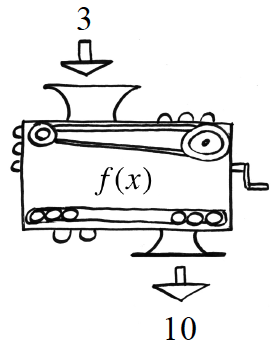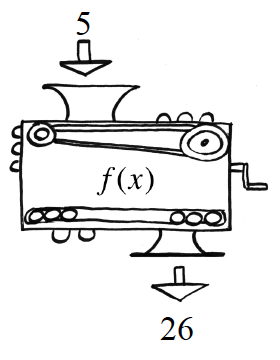### Home > A2C > Chapter 4 > Lesson 4.3.2 > Problem4-152

4-152.

Remember function machines? Each of the following pictures shows how the same machine changes the given $x$-value into a corresponding $f (x)$ value. Find the equation for this machine.Copy and complete the table for the function.

 x f(x) $10$ $2$ $3$ $101$ $5$

$f(x)=x^2+1$